STATICALLY INDETERMINATE PROBLEMS IN TENSION AND COMPRESSION

# 1STATICALLY INDETERMINATE PROBLEMS IN TENSION AND COMPRESSION

## 1.1Problem definition

Determinate the existing compression stress, when a double shell system with two different material is compressed by two vertical loads. Also, the negative elongation (unit compression) is verified.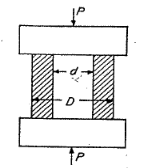Figure 2: Model by Timoshenko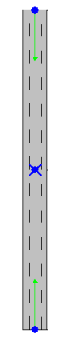Figure 1: Rohr2 model

## 1.2 References (Timoshenko)

S. Timoshenko, Strength of Material, Part I, Elementary Theory and Problems, 3rd Edition, D. Van Nostrand Co., Inc., New York, NY, 1956, Chapter 1.6, pg. 21-22.

System consists of two concentric, coupled beams submitted to compressive forces. The following parameters are given:

• Modulus of Elasticity (carbonsteel, copper)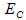,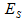• Compression load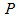• ratio of the E- Modulus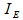• Diameter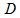,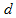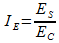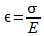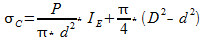Where:

 Variable Description Unit Used Value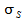Stresses in the steel beam lbs/ inchÂ² 3060,67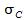Stresses in copper beam lbs/ inchÂ² 1632,36Modulus of Elasticity (Steel) lbs/ inchÂ² 30000000Modulus of Elasticity (Copper) lbs/ inchÂ² 16000000Ratio of E- Modulus 1,88Outside Diameter (Copper) inch 8,0Diameter (Steel) inch 4,0Compressive Load lbf 100000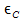Unit Compression (Copper) inch 0,000102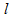Length ft/ inch 4,17/ 50,04

Table 1: Overview of the variables used

## 1.3 Model description (ROHR2)

The ROHR2 model consists of a double jacked line with full couplings at both ends. The length of the bars is 4,17 ft. They are made of two different materials. The first is a cylinder of carbon steel, the second part consists of copper (Pipe). This material has following mechanical parameters:

• Young's Modulus= 16 x 106 lbs/ inchÂ²

• Alfa T (68 Â°F)= 10,8 Î¼m/ m

• Tensile Strength= 28x 10Â³ lbs/ inchÂ²

The dimensions are taken from the example by Timoshenko. The copper tube has an outside diameter of eight inch. The inside diameter of the copper tube of four inch is equal to the outside diameter of the steel beam. An anchor point fixes the two parts at the middle and coupling are connecting the inner and outer parts at both ends. With this boundary conditions, it is guaranteed that the forces (100000 lbf) are transferred to both materials. The normal force is applied at the ends (P1a, P2a) and oriented towards the anchor point in the middle. The force were scaled by a factor at both model-versions (R005_inch: 1000; R005_mm: 10) at the lower ends, to increase the number of available digits in the output. As the gravitational acceleration should not be taken into account, an additional load case (occasional loads; Load1) is defined which contains only the two end forces.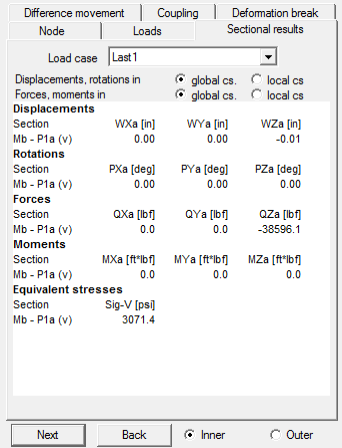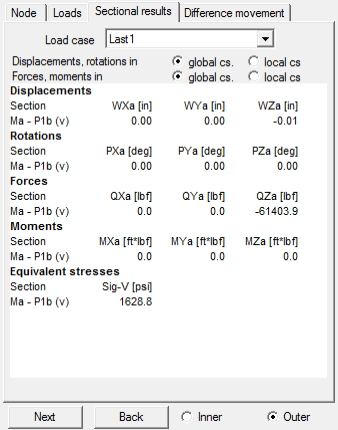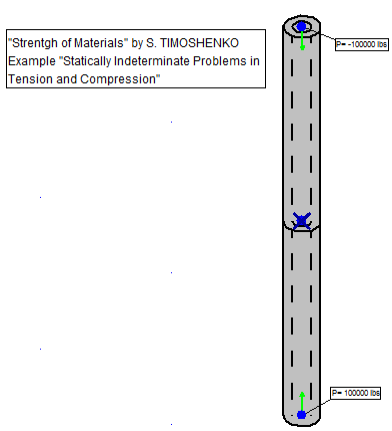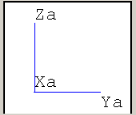Figure 3: Tension by P=100000 lbs (outer â‰™ Ïƒc; inner â‰™ Ïƒs)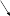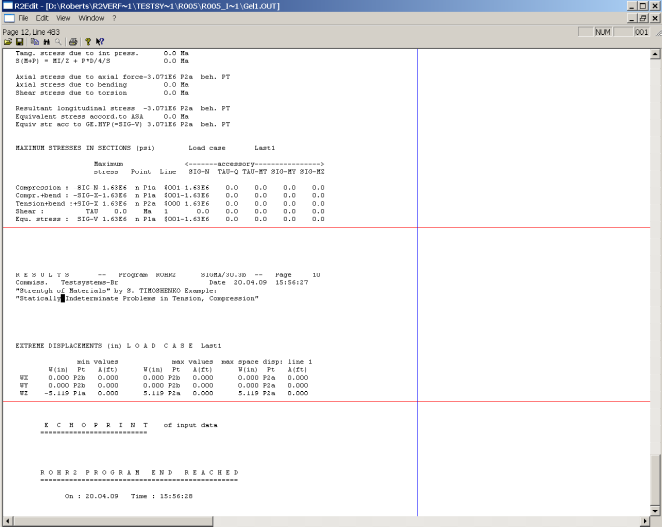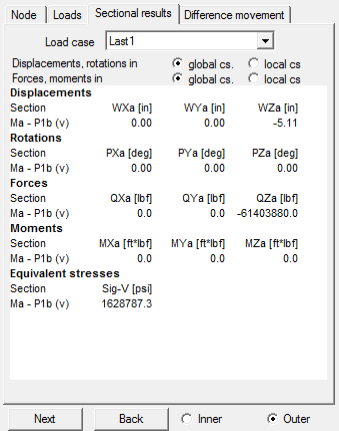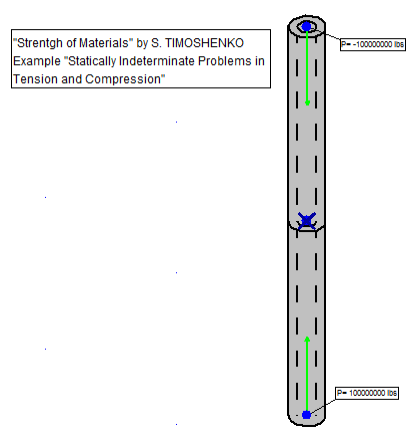Figure 4: Compression displacements by increased loads (outer â‰™ Îµc)

## 1.4 Result comparisons

 Value Length [inch] Reference (Timoshenko) [lbs/ inchÂ²] Rohr2 [lbs/ inchÂ²] Difference [%]50.04 3060,67 3071,4 <0,351632,36 1628,8 <0,22

Table 2: Comparison for the tension of both materials

 Value Length [inch] Reference (Timoshenko) [inch] Rohr2 [inch] Difference [%]50.04 0,005105 0,005119 <0,28

Table 3: Comparison of the unit compression for copper

## 1.5 Conclusion

The results are very close to the reference by Timoshenko. The difference are within the precision expected, given the number of digits in the input.

## 1.6 Files

R005_inch.r2w

R005_mm.r2w

R2_stresses_5.ods

MATDAT.r2u

SIGMA Ingenieurgesellschaft mbH www.rohr2.com